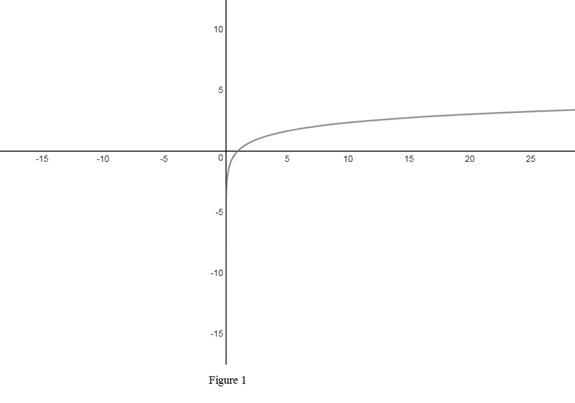# Whether the statement, if 0 &lt; a &lt; b then ln a &lt; ln b is true or false.### Single Variable Calculus: Concepts...

4th Edition
James Stewart
Publisher: Cengage Learning
ISBN: 9781337687805### Single Variable Calculus: Concepts...

4th Edition
James Stewart
Publisher: Cengage Learning
ISBN: 9781337687805

#### Solutions

Chapter 1, Problem 9RQ
To determine

## Whether the statement, if 0<a<b then lna<lnb is true or false.

Expert Solution

Solution: The statement is true.

### Explanation of Solution

Let the function be f(x)=lnx.

The graph of f(x)=lnx is shown below in Figure 1.From Figure 1, it is observed that the domain of the function is (0,) and the function f(x)=lnx is an increasing function.

Recall the definition of increasing function, “the function y=f(x) is said be an increasing function on the given interval [a, b] if f(x1)<f(x2) whenx1<x2, x1,x2(a,b)”.

It is given that, 0<a<b.

Then by the above definition, f(a)<f(b). That is, lna<lnb.

Therefore, the given statement is true.

### Have a homework question?

Subscribe to bartleby learn! Ask subject matter experts 30 homework questions each month. Plus, you’ll have access to millions of step-by-step textbook answers!